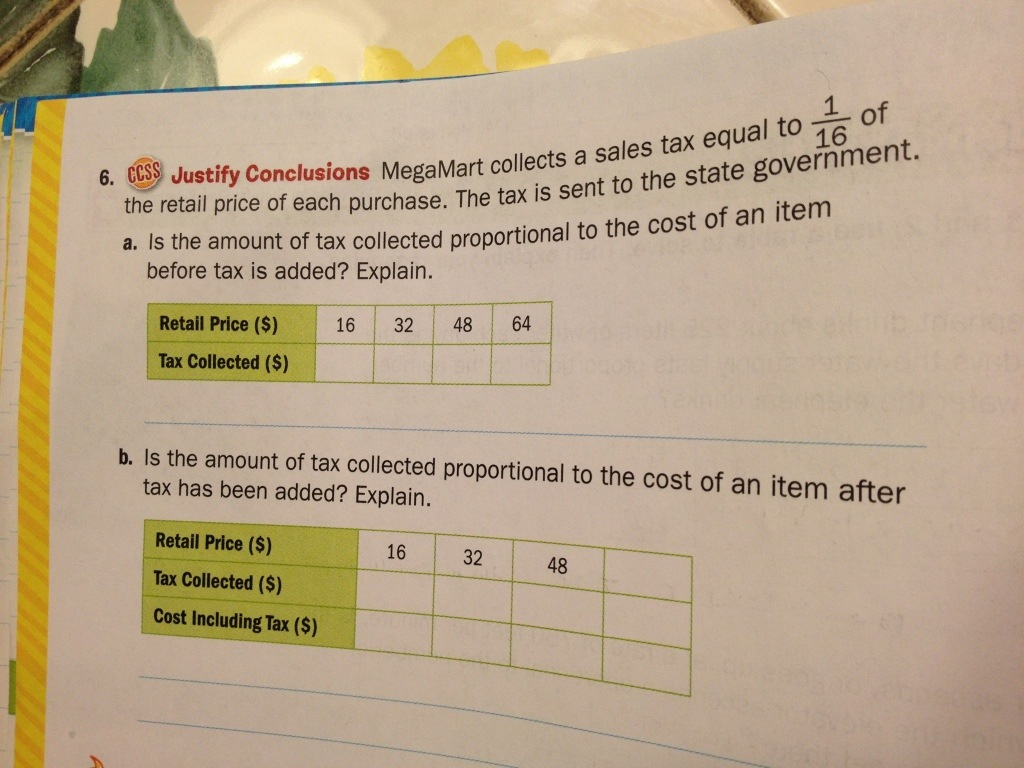# What common core math gets right

I posted earlier about finding out that my son’s 7th grade math workbook had been “infected” with common core (“your” common core no less…I didn’t ask for it, why is it mine?).
At first glance I wasn’t too worried about it.  That started to change a little last night at the dinner table when I was helping him with homework.  He’s been working on basic arithmetic with positive and negative numbers – adding negatives, subtracting a negatives from a positive, and multiplying strings of numbers with mixed signs.  About halfway through the homework assignment he got to one of my least favorite problem types: cartoon problems.  Yep, that’s right, his common core math workbook includes problems that are given in the form of comic book frames.
Putting the issue of how the problem was posed aside though, it was actually the content of this problem that really bothered me.  The problem was stated as:

You can sell a shirt with front and back printing for \$18.  It costs you 8\$ for the shirt, \$4 to print the back and \$3 to print the front.  If you decide to give away a shirt, how many shirts would you have to sell to make up for the lost profit?

Simple enough right?  We started by adding of all the part costs, to figure out a total cost (\$15).  Then we subtracted that from the sale price (\$18) to figure out the profit (\$3) on each sale.   We finished up by taking the \$15 and dividing it by \$3 to figure out that you would need to sell 5 shirts to cover the costs of giving one away.
But hold on a minute, the question was how many would we have to sell to make up for the lost profit.  I didn’t need to do any of that to answer that question.  In fact, I didn’t need to know any thing about the cost, the revenue or the profit to answer that question.  To make up for the profit I lose by giving away one of anything I need to sell….another one of the same thing (of course assuming the same cost and price).  By giving the shirt away I lose \$3 in potential profit.  To make that up, I need to sell another shirt, which nets me \$3.
This is either the trickiest math problem ever devised for 7th grade Math or (more likely) they meant to ask how many shorts would you have to sell to cover your costs.  My guess is that the folks that wrote my son’s common core math workbook really just don’t understand the concepts of cost, revenue and profit.  Of course they do get some things right….like taxes:Posted

in

by

Tags: